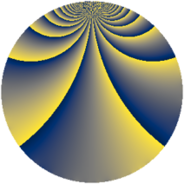# Properties

 Label 1045.2.yLevel $1045$ Weight $2$ Character orbit 1045.y Rep. character $\chi_{1045}(151,\cdot)$ Character field $\Q(\zeta_{10})$ Dimension $320$ Sturm bound $240$

# Related objects

## Defining parameters

 Level: $$N$$ $$=$$ $$1045 = 5 \cdot 11 \cdot 19$$ Weight: $$k$$ $$=$$ $$2$$ Character orbit: $$[\chi]$$ $$=$$ 1045.y (of order $$10$$ and degree $$4$$) Character conductor: $$\operatorname{cond}(\chi)$$ $$=$$ $$209$$ Character field: $$\Q(\zeta_{10})$$ Sturm bound: $$240$$

## Dimensions

The following table gives the dimensions of various subspaces of $$M_{2}(1045, [\chi])$$.

Total New Old
Modular forms 496 320 176
Cusp forms 464 320 144
Eisenstein series 32 0 32

## Trace form

 $$320 q - 80 q^{4} + 20 q^{6} + 56 q^{9} + O(q^{10})$$ $$320 q - 80 q^{4} + 20 q^{6} + 56 q^{9} + 8 q^{11} - 80 q^{16} - 40 q^{17} + 30 q^{19} + 32 q^{23} - 60 q^{24} - 80 q^{25} + 16 q^{26} + 148 q^{36} - 50 q^{38} + 60 q^{39} - 76 q^{42} + 36 q^{44} - 32 q^{47} + 116 q^{49} - 30 q^{57} - 104 q^{58} + 40 q^{61} + 40 q^{62} - 152 q^{64} - 8 q^{66} + 120 q^{68} + 40 q^{73} - 100 q^{74} + 36 q^{77} + 24 q^{80} - 276 q^{81} + 116 q^{82} + 40 q^{83} + 16 q^{92} - 48 q^{93} + 120 q^{96} + 96 q^{99} + O(q^{100})$$

## Decomposition of $$S_{2}^{\mathrm{new}}(1045, [\chi])$$ into newform subspaces

The newforms in this space have not yet been added to the LMFDB.

## Decomposition of $$S_{2}^{\mathrm{old}}(1045, [\chi])$$ into lower level spaces

$$S_{2}^{\mathrm{old}}(1045, [\chi]) \cong$$ $$S_{2}^{\mathrm{new}}(209, [\chi])$$$$^{\oplus 2}$$